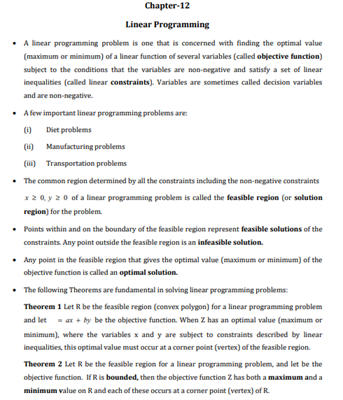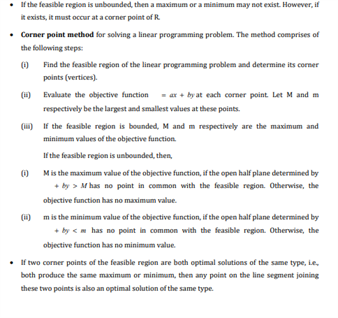# Maths Revision Notes for Class 12 Chapter 12 Linear Programming.

The 12th chapter for CBSE Class 12 Maths is titled as Linear Programming. In mathematics, it can simply be defined as the method used for minimizing or maximizing the linear function of several variables. With the help of these revision notes, students can make last-minute preparations while preparing for their math exams. Some of the various concepts that are introduced in this chapter are:

• Definition of Linear programming and its applications. It is primarily used in everyday life, for obtaining the minimum and maximum values which are used in making business decisions as well.
• Other important mathematical formulas that students are taught in this chapter are Optimal value, Objective function, Linear constraints, Non-negative restrictions and, Optimisation problems.
• Apart from this, the mathematical formulation of the problem is also provided, along with the graphical method used in solving linear programming problems.

You can easily find the revision notes PDF for chapter 12 Linear programming, for class 12 maths syllabus below:You may also want to read:

 NCERT Related Articles NCERT Solutions for Class 12 NCERT Solutions for Class 11 NCERT Solutions for Class 10 NCERT Solutions for Class 12 Maths NCERT Solutions for Class 11 Maths NCERT Solutions for Class 10 Maths NCERT Solutions for Class 12 Physics NCERT Solutions for Class 11 Physics NCERT Solutions for Class 10 Science NCERT Solutions for Class 12 Chemistry NCERT Solutions for Class 11 Chemistry NCERT Solutions for Class 6 NCERT Solutions for Class 12 Biology NCERT Solutions for Class 11 Biology NCERT Solutions for Class 6 Maths NCERT Solutions for Class 9 NCERT Solutions for Class 8 NCERT Solutions for Class 6 Science NCERT Solutions for Class 9 Maths NCERT Solutions for Class 8 Maths NCERT Solutions for Class 4 NCERT Solutions for Class 9 Science NCERT Solutions for Class 8 Science NCERT Solutions for Class 4 Maths NCERT Solutions for Class 5 NCERT Solutions for Class 7 NCERT Solutions for Class 7 Maths NCERT Solutions for Class 4 Science NCERT Solutions for Class 5 Maths NCERT Solutions for Class 7 Science NCERT Solutions NCERT Books NCERT Solutions for Class 5 Science